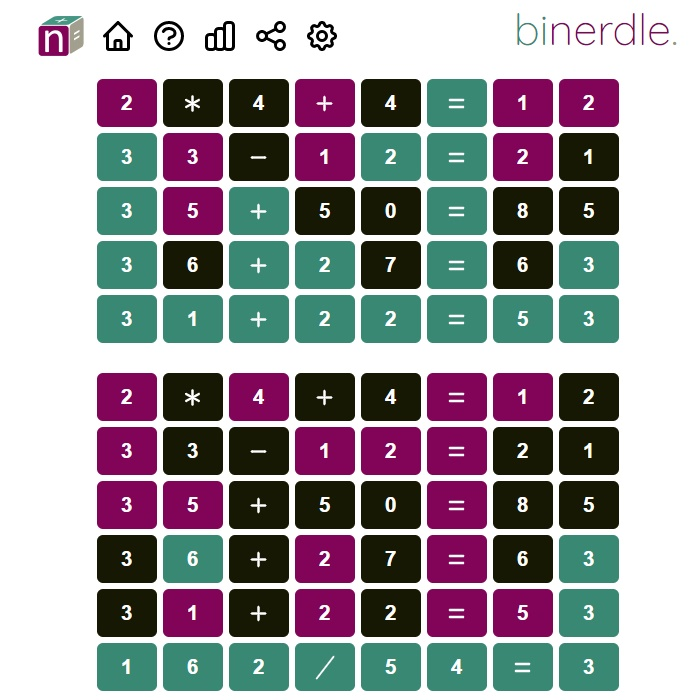Binerdle
In Binerdle, guess 2 NERDLEs in 7 attempts. Bi-Nerdle is like Quordle in that players have to solve multiple puzzles at the same time.Binerdle
13 3.04
Fullscreen

## Recommended for you

Wordle Unlimited» Binerdle

# Binerdle

Share

In Binerdle, guess 2 NERDLEs in 7 attempts. The color of each tile will change after each guess to indicate how close your guess was to the solution. Bi-Nerdle is like Quordle in that players have to solve multiple puzzles at the same time.

## How to play Binerdle

In Binerdle, players have to guess the whole correct equation. This means that to get the right answer, players have to figure out the math problem and how to solve it. They can do this by typing in numbers and mathematical symbols and then pressing the Enter button. The game tells you which choices are right and which ones are wrong.

## Rules

• Each guess is a kind of math.
• 0 1 2 3 4 5 6 7 8 9 + - * / or = can be used.
• It must have one "=" in it.
• To the right of the "=", there should only be a number, not another calculation.
• Remember learning about the "order of operations" in math class? Don't forget to figure out the answer by multiplying and dividing first. For example, 1+2*3 = 7 because it's the same as 1+6.
• If 10+20=30 is one of the answers we're looking for, we'll also accept 20+10=30, unless you turn off "commutative answers" in the settings.
• If, for example, your guess has two 1s but the answer only has one, you will get one colored tile and one black tile.

When a number is in the right place or when a full guess is rearranged as a winning commutative answer, the tiles will turn green. Binerdle is very hard because there are two puzzles going on at the same time. One good strategy is to try to solve one problem at a time and then move on to the next one once the first one has been solved. Once you've mastered the game, you can then go back to eight characters at any time. Have fun!

Comment (0)Be the first to comment